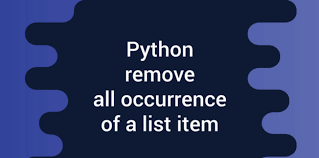# How to Remove All Occurrences of Value from List in Python

Python lists are powerful data structures that allow you store same or different data types in a compact manner. They also provide tons of functions and features making it a popular choice for python developers. While working with lists, you may need to remove all occurrences of value from list in Python. Remove() function removes only the first occurrence of value in list. In this article, we will learn how to remove all occurrences of value from list in python.

## How to Remove All Occurrences of Value from List in Python

Let us say you have the following list, and you want to remove all occurrences of 2 in it.

`a = [1, 2, 3, 4, 2, 2, 3]`

There are several ways to do this. We will look at some simple ones.

## 1. Using List Comprehension

You can easily remove all occurrences of value using list comprehensions as shown below.

```>>> [value for value in a if value != 2]
[1, 3, 4, 3]```

This is quite intuitive as we are basically iterating through the list items one by one and returning items that are not equal to 2.

You can even create a function out of the list comprehension.

```def remove_all_occurrences(the_list, val):
return [value for value in the_list if value != val]

print remove_all_occurrences(a, 2)
[1, 3, 4, 3]
```

Also this method does not modify the original list but returns a new one. If you want to modify the original list, you will need to slightly modify the above list comprehension to use slice notation.

```>>> a = [1, 2, 3, 4, 2, 2, 3]
>>> a[:] = (value for value in a if value != 2)
>>> a
[1, 3, 4, 3]```

## 2. Using filter+lambda

You can also use a combination of filter and lambda to remove all occurrences of item from list. Here are a couple of examples for python 3.x

```>>> a = [1,2,3,2,2,2,3,4]
>>> list(filter((2).__ne__, a))
[1, 3, 3, 4]

OR

>>> a = [1,2,3,2,2,2,3,4]
>>> list(filter(lambda a: a != 2, a))
[1, 3, 3, 4]
```

If you are using python 2.x then you need to use the following filter function.

```>>> a = [1,2,3,2,2,2,3,4]
>>> filter(lambda a: a != 2, a)
[1, 3, 3, 4]```

Filter function filters the given list using lambda function that tests each item of the list to be true or not. In our case, the test is to check if the given element is not equal to 2. So all the above filter functions check each item of the list one by one, to see if they are not equal to 2, and return only those elements who return true for this test.

In this article, we have learnt a couple of ways to remove all occurrences of element from list. It is advisable to use the first method since it is intuitive, faster and more efficient than the second one.